by PALAKOLLU SRI MANIKANTA

In this article, you will get a deep understanding of functional programming and its benefits.

Introduction To Functional Programming

Functional programming (FP) is a type of paradigm or pattern in computer science. Everything is done with the help of functions in FP and the basic building blocks are functions only.

Programming languages that support purely functional programming are —

2. Closure
3. Scala
4. SQL

Some of the programming languages that support functional programming as well as other programming paradigms are —

1. Python
2. Javascript
3. C++
4. Ruby

Since the name says functional, most of the programmers think about Mathematical functions. That is not the case with FP. It is just an abstraction to solve real-world complex problems in an easy and effective manner.

Before the Object-Oriented programming Era, the software industry completely depended on functional programming. This paradigm rocked the software industry for a couple of decades. There are some issues with functional programming, and that’s why they moved to Object-Oriented paradigm. The issues with FP will be discussed later in this article.

That is all about the introduction to Functional Programming. Now, first of all, we need to learn what is a function.

Functions

Before revealing the actual definition, I want to explain a situation to know where to actually use FP. Suppose you are writing code to build an application. In your development journey, you want to reuse the code of a few lines (100) at different places. For your Application, functions are helpful. We can write functions at one place and we will be able to access those functions from anywhere in the program. Functional programming has the following features —

1. Reduces code redundancy.
2. Improves modularity.
3. Helps us to solve complex problems.
4. Increases maintainability.

Let’s look at the actual definition of a function:

A Function is a specified block of code which is used to perform a specific task in the program.

The most popular types of functions are —

1. General Functions
2. Arrow Functions
3. Anonymous Functions

General Functions

General functions are nothing but the functions that are quite often used by the programmer to perform a specific task. The syntax to declare a general function in Javascript is:

function functionName(parameters) {  // code to be executed}

function — It is a keyword which is necessary to declare a function.

functionName — It can be named based on the function work.

parameters — We can pass any number of parameters to a function.

Declared functions are not executed immediately. They are “saved for later use”, and will be executed later, when they are invoked (called upon).

We need to call the function when we want to execute that piece of code that is returned within a function.

The general functions are classified as follows —

No-Argument Functions

We don’t need to pass any arguments to the function.

// Function Declaration
// Calling the functionsayHello()

When we call the function to sayHello() it will produce the alert message as Hello.

Argument Functions

In this type of functions, we will pass arguments to them.

Example

// Declaring a Function
function add(num1, num2){   return num1 + num2;}
// Function Call
console.log(result);

The arguments that are passed while declaring a function i.e (num1, num2) are called as Formal Parameters.

The arguments that are passed while calling a function i.e (7, 11) are called as Actual Parameters.

A Function usually returns some value, and to return that value we need to use return keyword. When a function is returning some value it means it doesn’t print any output for us, it just returns the final output. It is our responsibility to print that result. In the above program, the function is returning the value and I’m passing that value to a variable name ‘result’. Now the function will pass the result to the ‘result’ variable.

The speciality of Javascript Functions

If you pass more arguments than the declared number, then you will not get any error. But in other programming languages like Python, C, C++, Java, etc… we will get an error. Javascript will consider based on their requirements.

Example

// Calling the function with more number of arguments than the declared number
var result1 = add(2, 4, 6);console.log(result1);

OutputOutput and Execution of the above program in chrome Javascript console.

If you pass fewer arguments than the declared number, then also we will not get any error. But we can’t predict the output for the program because, based on your function functionality, the output will be produced.

Variable Argument Function

The greatest advantage of Javascript functions is we can pass any number of arguments to the function. This feature helps developers to work more effectively in a consistent manner.

Example

// Creating a function to calculate sum of all argument numbers
function sumAll(){
let sum = 0;
for(let i=0;i<arguments.length;i++){      sum = sum + arguments[i];}
return sum;
}
// Calling the sumAll function
sumAll();
sumAll(1,2,3,12,134,3234,4233,12,3243);

Output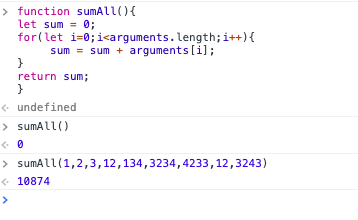Output and Execution part of the above program in chrome Javascript console.

This is all about general functions that are used to perform our complex task in a simple manner. Now let’s discuss some advanced functions introduced in ES6 called Arrow Functions.

Arrow Functions

An arrow function expression is a syntactically compact alternative to a regular function expression. It doesn’t have its own bindings to the this, super, arguments or new.target keywords. Arrow function expressions are ill-suited as methods. They cannot be used as constructors.

One of the most loved features in Es6 are Arrow functions. This arrow function helps developers time and simplify function scope.

The syntax for the arrow function is:

const functionName = (parameters) => {  // code to be executed}
(OR)
var functionName = (parameters) => {  // code to be executed}
(OR)
let functionName = (parameters) => {  // code to be executed}

Examples for Arrow Functions

Eg 1

Creating an Arrow function to say a welcome message to the users.

// Creating a Welcome function
let sayHello = () => {   return 'Welcome to Javascript World...!';}
// Calling the function
console.log(sayHello())

OutputOutput and Execution part of the above program in chrome Javascript console.

Eg 2

In this example, we are creating an Arrow function to generate the greatest of all numbers that are passed as an argument.

let maxNumber = (a,b,c,d) => {
if(a > b && a > c && a > d)       return a;   else if(b > a && b > c && b>d)       return b;   else if(c > a && c > b && c > d)       return c;   else       return d;}
// Calling the function
console.log(maxNumber(1,2,4,3));

Output: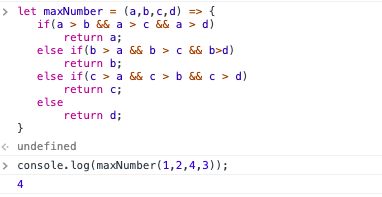Output and Execution part of the above program in chrome Javascript console.

Combination of Variable Arguments with Arrow Functions

Since we are working with an arrow function, it doesn’t support the arguments array by default like general function. It is our responsibility to declare explicitly that it supports the variable number of arguments

Eg 3

let varArgSum = (...args) => {   let sum = 0;
for(let i=0;i<args.length;i++){      sum = sum + args[i];}
return sum;
}
// Calling the Function
console.log(varArgSum());
console.log(varArgSum(1,2,3,4,5,6,7,8,9,10));

Output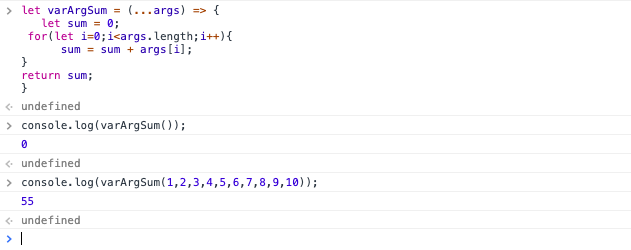Output and Execution part of the above program in chrome Javascript console.

This is how we can combine a variable number of arguments with arrow functions. Now let’s discuss Anonymous functions in JavaScript.

Anonymous Functions

An anonymous function is simply a function with no name. The purpose of using anonymous function is to perform a certain task and that task is no longer required to program. Generally, anonymous functions are declared dynamically at run time.

Anonymous functions are called only once in a program.

Example:

// Working with an Anonymous function
var a = 10;  // Global Scope Variable.
// creating a function(function() {
console.log("welcome to the world of Anonymous function");
var b = 20;  // b is a local scope variable.
var c = a+b; // c is a local scope variable    //a can be used because it is in the global scope
console.log("Addition of two numbers value is: "+c);})();

Output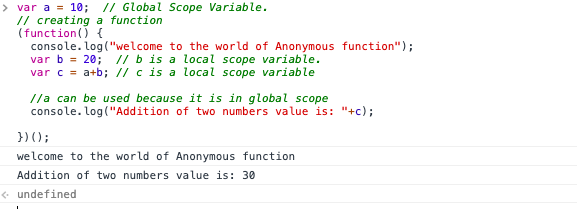Output and Execution part of the above program in chrome Javascript console.

This is the concept of anonymous functions. I think I explained it in a simple and easy way.

Higher Order Functions

A higher-order function is a function that takes functions as an argument or that returns another function as a result.

The best example of higher-order functions in Javascript is that of Array.map(), Array.reduce(), Array.filter().

Example 1: Array.map()

// working with Array.map()
let myNumberArray = [4,9,16,25,36,49];
let mySquareRootArray = myNumberArray.map(Math.sqrt);
console.log(mySquareRootArray);

OutputOutput and Execution part of the above program in chrome Javascript console.

Example 2: Array.reduce()

// working with Array.reduce()
let someRandomNumbers = [24,1,23,78,93,47,86];
let newReducedResult = someRandomNumbers.reduce(getSum);
console.log(newReducedResult);

Output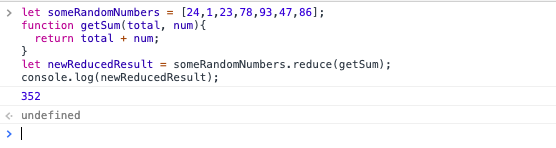Output and Execution part of the above program in chrome Javascript console.

Example 3: Array.filter()

// Working with array filter
let ages = [12,24,43,57,18,90,43,36,92,11,3,4,8,9,9,15,16,14];
function rightToVote(age){   return age >= 18;}
let votersArray = ages.filter(rightToVote);
console.log(votersArray);

Output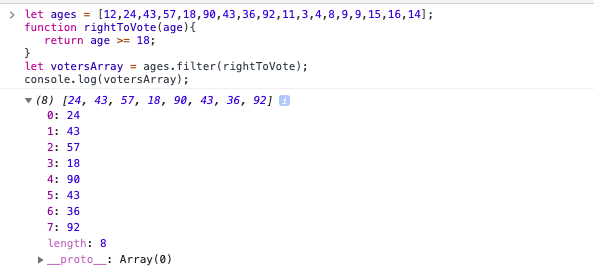Output and Execution part of the above program in chrome Javascript console.

Recursion

This is one of the key topics in functional programming. The process in which a function calls directly or indirectly is called a recursive function. This concept of recursion is quite useful in solving algorithmic problems like the Towers of Hanoi, Pre-Order, Post-Order, In-Order, and some graph traversal problems.

Example

Let’s discuss a famous example: finding the factorial of a number using recursion. This can be done by calling the function directly from the program repeatedly. The logic for the program is

factorial(n) = factorial(n) * factorial(n - 1) * factorial(n - 2) * factorial(n - 3) * ….. * factorial(n - n);
// Finding the factorial of a number using Recursion
function factorial(num){  if(num == 0)        return 1;  else        return num * factorial(num - 1);
}
// calling the function
console.log(factorial(3));
console.log(factorial(7));
console.log(factorial(0));

Output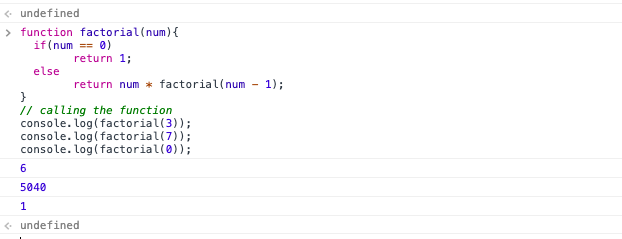Output and Execution part of the above program in chrome Javascript console.

Characteristics Of Functional Programming

The objective of any FP language is to mimic the use of mathematical concepts. However, the basic process of computation is different in functional programming. The major characteristics of functional programming are:

Data is immutable: The data which is present inside the functions are immutable. In Functional programming, we can easily create a new Data structure but we can’t modify the existing one.

Maintainability: Functional programming produces great maintainability for developers and programmers. We don’t need to worry about changes that are accidentally done outside the given function.

Modularity: This is one of the most important characteristics of functional programming. This helps us to break down a large project into simpler modules. These modules can be tested separately which helps you to reduce the time spent on unit testing and debugging.

1. It helps us to solve problems effectively in a simpler way.
2. It improves modularity.
3. It allows us to implement lambda calculus in our program to solve complex problems.
4. Some programming languages support nested functions which improve maintainability of the code.
5. It reduces complex problems into simple pieces.
6. It improves the productivity of the developer.
7. It helps us to debug the code quickly.

1. For beginners, it is difficult to understand. So it is not a beginner friendly paradigm approach for new programmers.
2. Maintainance is difficult during the coding phase when the project size is large.
3. Reusability in Functional programming is a tricky task for developers.

Conclusion

For some, it might be a completely new programming paradigm. I hope you will give it a chance in your programming journey. I think you’ll find your programs easier to read and debug.

This Functional programming concept might be tricky and tough for you. Even if you are a beginner, it will eventually become easier. Then you can enjoy the features of functional programming.